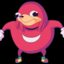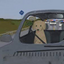MIT 6.00 Intro Computer Science (OCW)OpenStudy (anonymous):

Problem set 2, problem 4OpenStudy (anonymous):

I'm having trouble with problem four. For problem three, I exploited the fact that because the GCD of 6 and 9 is 3 and because 6 and 9 are three apart, any multiple of 3 > 6 is a buyable number of mcnuggets. And if the number is greater than 20, you can just subtract twenty and repeat that test. But for problem four, I'm trying to write a program that asks the user for the values of x, y, and z. Basically, it starts by asking you to set the values of the small, medium, and large sizes of mcnuggets, then proceeds to do the same test. At least, I'd like it to perform the same test. Can any advise me on a mathematical strategy/method for how solve a diophantine equation? I'm fine with doing an "exhaustive search" as the instructions say, basically looking at every possible combination of x, y, and z, but I can't figure our a way to represent that logically. Make sense?OpenStudy (anonymous):

I used a series of nested loops to test various quantities of smalls, mediums, and largesOpenStudy (anonymous):

The thing that I used to limit the loop was the total number of possible McNuggets--the problem says "find the largest number (less than 200) of McNuggets that cannot be bought in exact quantity," so if you get up to 200, you can stop. Then for me the relevant question became "If I can test total numbers of McNuggets up to a certain number, how can I test each possible combination of size-quantities that might total that number of McNuggets?"

Latest Questionsowenjiang: determine whether the graph represents a proportional relationship
7 minutes ago 0 Replies 0 MedalsToxicaDennisIce: Which number completes the inequality? 1.01 less-than blank less-than 1.17, less-than 1.
59 seconds ago 42 Replies 3 Medalsyesno: um, sorry for asking for so much help but... can i get more? if so, thanks. QUESTION 4 At the pet fair, there are 42 dogs and 35 cats.
7 minutes ago 10 Replies 2 Medalsyesno: Omariyah, you can still work on the question. if you need it again here it is. QUESTION 1 Find the GCF of the numbers.
15 minutes ago 4 Replies 0 MedalsToxicaDennisIce: Which list correctly orders A, B, and C from least to greatest when A = StartAbso
16 minutes ago 12 Replies 0 Medalskarlidene: How high was the plane after 100010001000 seconds?
6 minutes ago 4 Replies 0 Medalssupie: Find the x-intercept of the rational function. A rational function is graphed in the second quadrant, and in the first, third and fourth quadrants are other
19 minutes ago 6 Replies 2 Medalsyesno: help? Find the GCF of the numbers. 50, 40 A. Make sure to include both factor lists to show how you arrived at your answer (your work).
17 minutes ago 74 Replies 4 MedalsToxicaDennisIce: Which represents the value of Point C on the number line? A number line has points blank, A, negative 2, B, 0, C, 2, blank, D.
24 minutes ago 4 Replies 0 MedalsMeme69420: Which existing technology did engineers use to enhance the speed of propeller-dri
17 minutes ago 12 Replies 1 Medal